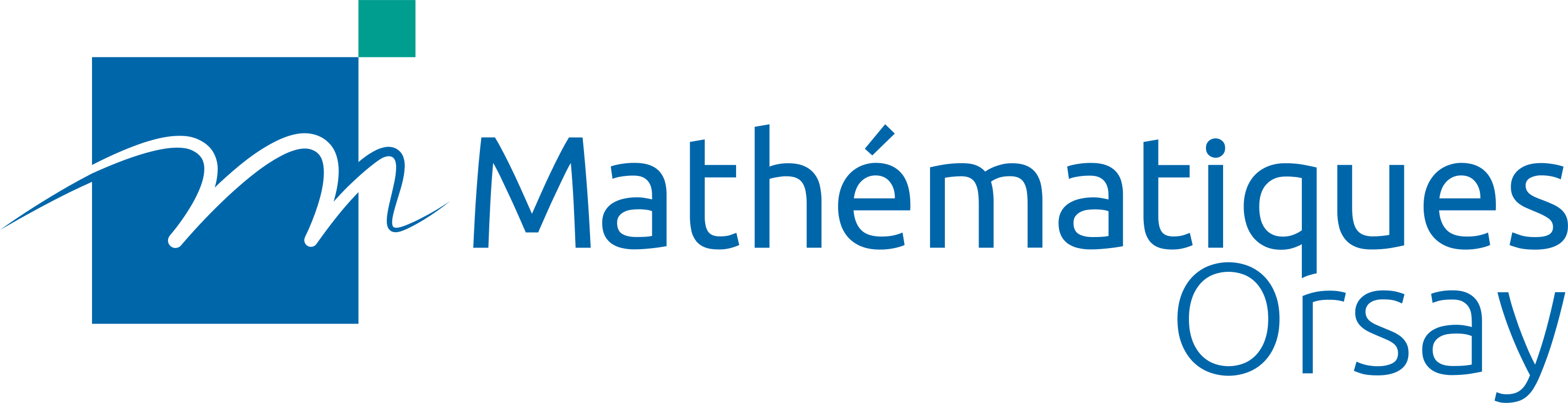# Matthew Morrow

Directeur de recherche, CNRS

Laboratoire de Mathématiques d’Orsay
Bâtiment 307, rue Michel Magat
Faculté des Sciences d’Orsay, Université Paris-Saclay
F-91405 Orsay Cedex

Office: LMO, room 3H20.

firstname.lastname@universite-paris-saclay.fr

### Research interests

Algebraic K-theory, arithmetic geometry, (topological) cyclic homology, algebraic cycles, p-adic Hodge theory.

### ERC projet MoCoS

My research is funded by the ERC Consolidator Grant 101001474 Motivic Cohomology of Schemes (MoCoS), 1 September 2021 – 31 August 2026.
The current project team consists of Matthew Morrow (PI), Ryomei Iwasa (postdoc), Hyungseop Kim (postdoc), Tess Bouis (PhD student), and Hari Sudarsan (PhD student).
Former members: Elden Elmanto (postdoc).

### Preprints

• Motivic cohomology of equicharacteristic schemes, with Elden Elmanto, arXiv, video (from Motives in Tokyo, February 2023)
• Generalised representations as q-connections in integral p-adic Hodge theory, with Takeshi Tsuji, arXiv
• p-adic vanishing cycles as Frobenius-fixed points, pdf

### Publications

1. Milnor K-theory of p-adic rings, with Morten Lüders pdf
Journal für die reine und angewandte Mathematik (Crelle's Journal), vol. 2023, no. 796, 69--116, 2023.
2. K-theory of perfectoid rings, with Benjamin Antieau and Akhil Mathew arXiv
Documenta Mathematica, 27, 1923--1951, 2022.
3. On the Beilinson fiber square, with Benjamin Antieau, Akhil Mathew, and Thomas Nikolaus pdf
Duke Mathematical Journal, to appear.
4. K-theory of valuation rings, with Shane Kelly pdf
Compositio Mathematica, vol. 157, issue 6, 1121--1142, 2021.
5. K-theory and topological cyclic homology of Henselian pairs, with Dustin Clausen and Akhil Mathew pdf
Journal of the American Mathematical Society, vol. 34, 411--473, 2021.
6. Topological Hochschild homology and integral p-adic Hodge theory, with Bhargav Bhatt and Peter Scholze pdf
Publications Mathématiques de l'IHÉS.
7. Integral p-adic Hodge theory, with Bhargav Bhatt and Peter Scholze pdf link
Publications Mathématiques de l'IHÉS.
8. K-theory and logarithmic Hodge-Witt sheaves of formal schemes in characteristic p arXiv
Annales Scientifiques de l'École Normale Supérieure, to appear.
9. A Variational Tate Conjecture in crystalline cohomology pdf
(with appendix A note on higher direct images in crystalline cohomology pdf)
Journal of the European Mathematical Society, to appear.
10. Analogues of Gersten's conjecture for singular schemes, with Amalendu Krishna pdf link
Selecta Mathematica, vol. 23, issue 2, 1235–1247, 2017.
11. Finite generation and continuity of topological Hochschild and cyclic homology, with Bjørn Ian Dundas pdf
Annales Scientifiques de l'École Normale Supérieure, vol. 50, fascicule 1, 201–238, 2017.
12. Integral p-adic Hodge theory – announcement, with Bhargav Bhatt and Peter Scholze pdf link
Mathematical Research Letters, vol. 22, number 6, 1601–1612, 2015.
13. Zero cycles on singular varieties and their desingularisations pdf link
Documenta Mathematica, extra volume dedicated to A. Merkurjev.
14. Pro unitality and pro excision in algebraic K-theory and cyclic homology pdf link
Journal für die reine und angewandte Mathematik (Crelle's Journal), to appear.
15. A case of the deformational Hodge conjecture via a pro Hochschild–Kostant–Rosenberg theorem pdf link
Comptes Rendus Mathématique, vol. 352, issue 03, 173–177, 2014.
16. Pro cdh-descent for cyclic homology and K-theory pdf link
Journal de l'Institut de Mathématiques de Jussieu, vol. 15, issue 03, 539–567, 2016.
17. K-theory of one-dimensional rings via pro-excision pdf link
Journal de l'Institut de Mathématiques de Jussieu, vol. 13, issue 02, 225–272, 2014.
18. A singular analogue of Gersten's conjecture and applications to K-theoretic adèles pdf link
Communications in Algebra, vol. 43, issue 11, 4951–4983, 2015.
19. K_2 of localisations of local rings pdf link
Journal of Algebra, vol. 399C, 190–204, 2014.
20. Continuity of the norm map on Milnor K-theory: a local proof pdf link
Journal of K-Theory, vol. 9, issue 03, 565–577, 2012.
21. Grothendieck's trace map for arithmetic surfaces via residues and higher adèles pdf link
Journal of Algebra and Number Theory, vol. 6, no. 7, 1503–1536, 2012.
22. An explicit approach to residues on and dualizing sheaves of arithmetic surfaces link
New York Journal of Mathematics, vol. 16, 2010.
23. Integration on valuation fields over local fields pdf
The Tokyo Journal of Mathematics, vol. 33, 2010, 235–281.
24. Integration on product spaces and GL_n of a valuation field over a local field link
Communications in Number Theory and Physics, vol. 2, no. 3, 563–592, 2008.

### Notes, etc.

• Raconte-moi... la courbe de Fargues–Fontaine pdf
La Gazette des mathématiciens 163 (January 2020).
• Topological Hochschild homology in arithmetic geometry link
2019 Arizona Winter School.
• The Fargues–Fontaine curve and diamonds [d'après Fargues, Fontaine, et Scholze] pdf video
Séminaire N. Bourbaki, juin 2018, exposé 1150.
• Notes on Ainf-cohomology pdf
A detailed overview of the construction of the Ainf-cohomology theory of the preprint Integral p-adic Hodge Theory with Bhargav Bhatt and Peter Scholze.
• The relative Fargues–Fontaine curve pdf
Oberwolfach report, Arbeitsgemeinschaft: The Geometric Langlands Conjecture (April 2016).
• A historical overview of pro cdh descent in algebraic K-theory and its relation to rigid analytic varieties arXiv
• Two questions on pro Milnor K-theory in characteristic p pdf
Oberwolfach report, Workshop: Algebraic K-theory and Motivic Cohomology (June 2016).
• An introduction to higher dimensional local fields pdf
An elementary introduction to the theories of higher dimensional local fields and higher dimensional adèles.

### Old teaching notes

• Specialised M2 course : Adic and perfectoid spaces [offline]
• MATH 242 pdf
Complete notes for MATH 242, Algebraic Number Theory, at the University of Chicago.

### Students